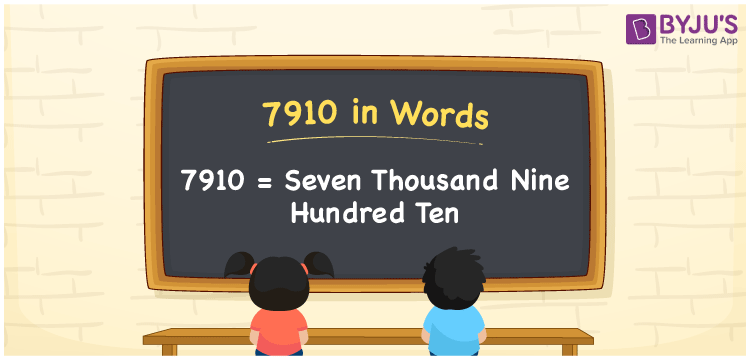# 7910 in Words

We can write 7910 in words as Seven thousand nine hundred ten. If you bought a new pair of shoes at Rs. 7910, then you can say, “I bought a new pair of shoes for Seven thousand nine hundred ten rupees”. In this article, you will learn how to convert the number 7910 to a word form with the help of a place value chart.

 7910 in words Seven thousand nine hundred ten Seven thousand nine hundred ten in Numbers 7910

## 7910 in English Words

We generally write numbers in words using the English alphabet. So, we can spell 7910 in English as “Seven thousand nine hundred ten”.## How to Write 7910 in Words?

The number 7910 has four digits, so we need to create a place value chart with four columns to specify the place values for all the digits of this number. This can be done as shown below.

 Thousands Hundreds Tens Ones 7 9 1 0

Here, ones = 0, tens = 1, hundreds = 9, and thousands = 7.

Thus, we can write the expanded form for the above digits as:

7 × Thousand + 9 × Hundred + 1 × Ten + 0 × One

= 7 × 1000 + 9 × 100 + 1 × 10 + 0 × 1

= 7000 + 900 + 10

= Seven thousand + Nine hundred + Ten

= Seven thousand nine hundred ten

Therefore, 7910 in words = Seven thousand nine hundred ten.

7910 is a natural number that precedes 7911 and succeeds 7909.

7910 in words – Seven thousand nine hundred ten

Is 7910 an odd number? – No

Is 7910 an even number? – Yes

Is 7910 a prime number? – No

Is 7910 a composite number? – Yes

Is 7910 a perfect square number? – No

Is 7910 a perfect cube number? – No

## Frequently Asked Questions on 7910 in Words

Q1

### How to write 7910 in words?

The number 7910 can be written in words as Seven thousand nine hundred ten.
Q2

### How do you write Rs. 7910 in words on a cheque?

On a cheque, an amount of Rs. 7910 can be expressed in words as “Seven thousand nine hundred ten rupees only”.
Q3

### What is the number name for 7910?

The number name for 7910 is Seven thousand nine hundred ten, i.e. 7910 = Seven thousand nine hundred ten.